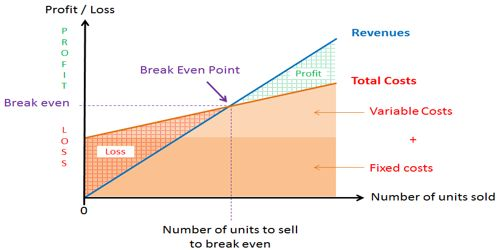# Why do you Analyze Break-even Point?

Break-even point is that level of operation at which sales revenues for a period are equal to the costs assigned to that period. As a result, there is no net profit or loss. An increase in the income tax rate does not affect the breakeven point. Operating income at the breakeven point is zero, and no income taxes are paid at this point. Simply, the breakeven point is; Total fixed costs / (Selling price – total variable costs).

The purpose of break-even analysis is to provide a rough indicator of the earnings impact of a marketing activity. The break-even point is one of the simplest yet least used analytical tools in management. The break-even point represents the level of sales revenue that equals the total of the variable and fixed costs for a given volume of output at a particular capacity use rate. It helps to provide a dynamic view of the relationships between sales, costs, and profits. However, there is an easy procedure called a break-even analysis that helps you realize how profits change as revenues vary. It is a useful tool for forecasting your break-even point in any given situation. For example, expressing break-even sales as a percentage of actual sales can give managers a chance to understand when to expect to break even (by linking the percent to when in the week/month this percent of sales might occur). This is very important to fix financial analysis.